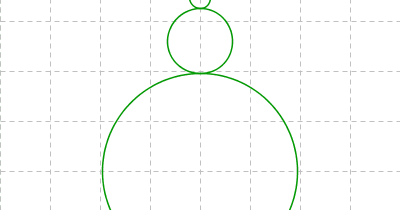# Fun math art (pictures) - benice equation

submited by
Style Pass
2023-11-19 20:30:07

The ratios between the size of the various circles set the limits of the function/expression, and being circular makes the results finite, so the end result is a fractal set, correct?

Hi Mr. Chen, I am a high school student studying computer science. These images have inspired me to create a program which simulates the patterns in them based on the following user inputted modifiers: number of circles, circle speed multiplier, circle size divisor, and circle direction (same or opposite). I have only created this program for individual educational purposes and will give you full credit when submitting it to my teacher. I have had no trouble creating this program, but at this point I would like to display the parametric equations of the pattern based on the user inputted modifiers. Is there any general parametric equation based on the inputted modifiers which can model these patterns? Thank you in advance, Jonathan

Hi SabayonCompiz, The most general parametric equation is: x(t) = Σ(k=1 to n) R(k)*cos(a(k)*t) y(t) = Σ(k=1 to n) R(k)*sin(a(k)*t) Here's an example in Graph: http://forum.padowan.dk/viewtopic.php?id=721